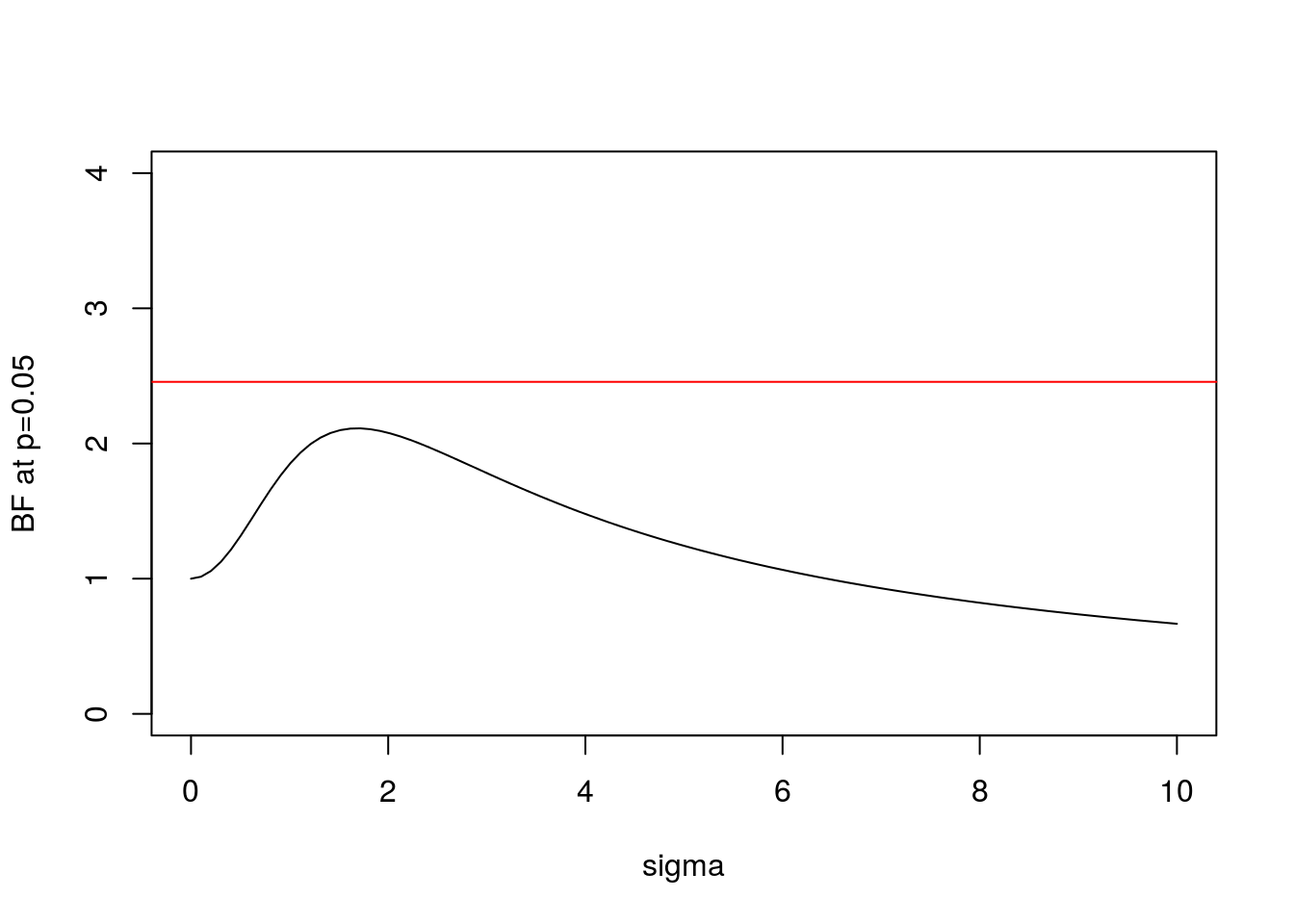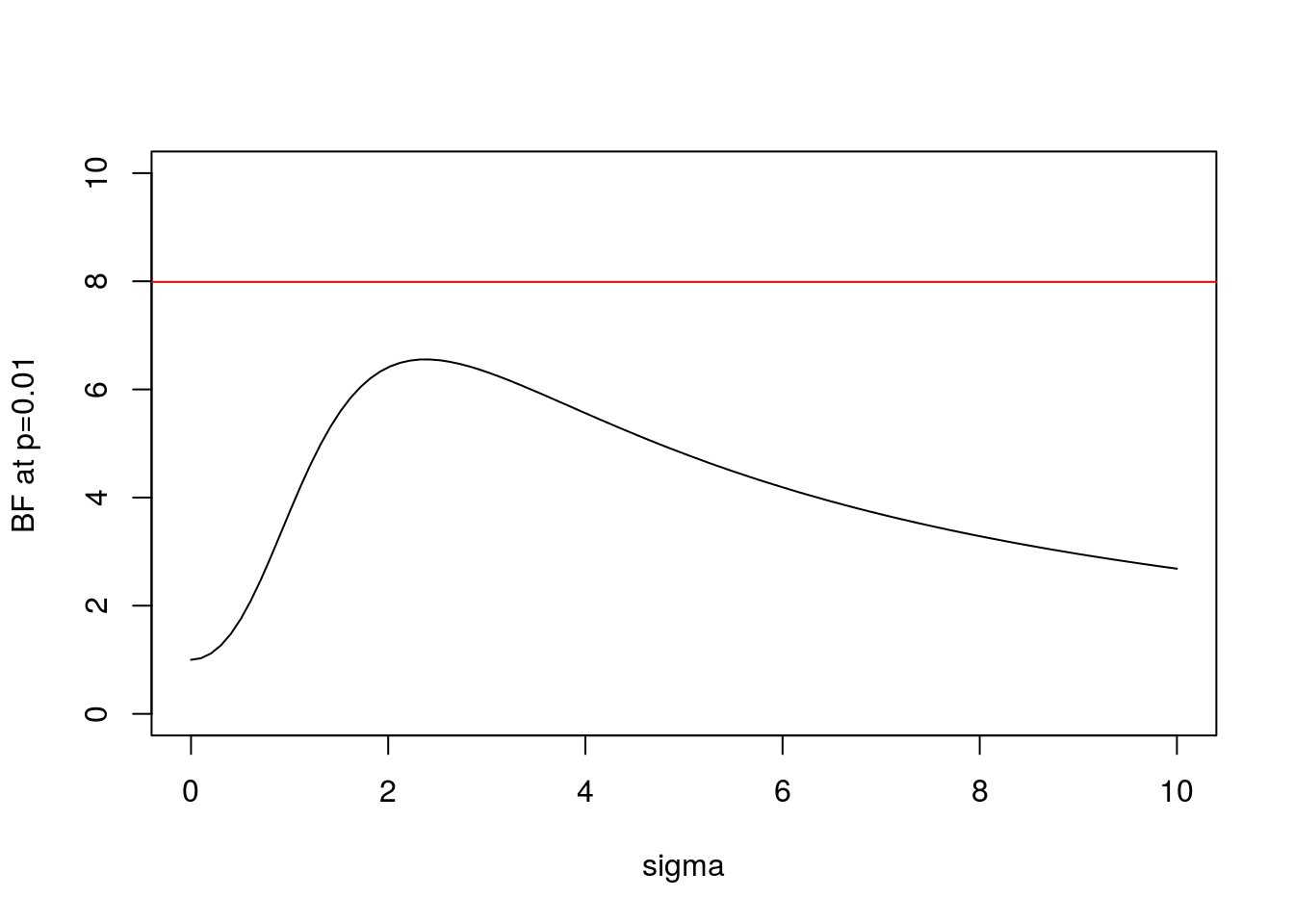Last updated: 2017-03-06

Code version: c7339fc

# Pre-requisites

You should know what a Bayes Factor is and what a p value is.

# Overview

Sellke et al (Thomas Sellke 2001) study the following question (paraphrased and shortened here).

Consider the situation in which experimental drugs D1; D2; D3; etc are to be tested. Each test will be thought of as completely independent; we simply have a series of tests so that we can explore the frequentist properties of p values. In each test, the following hypotheses are to be tested: $H_0 : D_i \text{ has negligible effect}$ versus $H_1 : D_i \text{ has a non-negligible effect}.$

Suppose that one of these tests results in a p value $$\approx p$$. The question we consider is: How strong is the evidence that the drug in question has a non-negligible effect?

The answer to this question has to depend on the distribution of effects under $$H_1$$. However, Sellke et al derive a bound for the Bayes Factor. Specifically they show that, provided $$p<1/e$$, the BF in favor of $$H_1$$ is not larger than $1/B(p) = -[e p \log(p)]^{-1}.$ (Note: the inverse comes from the fact that here we consider the BF in favor of $$H_1$$, whereas Sellke et al consider the BF in favor of H_0).

Here we illustrate this result using Bayes Theorem to do calculations under a simple scenario.

# Details

Let $$\theta_i$$ denote the effect of drug $$D_i$$. We will translate the null $$H_0$$ above to mean $$\theta_i=0$$. We will also make an assumption that the effects of the non-null drugs are normally distributed $$N(0,\sigma^2)$$, where the value of $$\sigma$$ determines how different the typical effect is from 0.

Thus we have: $H_{0i}: \theta_i = 0$ $H_{1i}: \theta_i \sim N(0,\sigma^2)$.

In addition we will assume that we have data (e.g. the results of a drug trial) that give us imperfect information about $$\theta$$. Specifically we assume $$X_i | \theta_i \sim N(\theta_i,1)$$. This implies that: $X_i | H_{0i} \sim N(0,1)$ $X_i | H_{1i} \sim N(0,1+\sigma^2)$

Consequently the Bayes Factor (BF) comparing $$H_1$$ vs $$H_0$$ can be computed as follows:

BF= function(x,s){dnorm(x,0,sqrt(s^2+1))/dnorm(x,0,1)}

Of course the BF depends both on the data $$x$$ and the choice of $$\sigma$$ (here s in the code).

We can plot this BF for $$x=1.96$$ (which is the value for which $$p=0.05$$):

s = seq(0,10,length=100)
plot(s,BF(1.96,s),xlab="sigma",ylab="BF at p=0.05",type="l",ylim=c(0,4))
BFbound=function(p){1/(-exp(1)*p*log(p))}
abline(h=BFbound(0.05),col=2)Here the horizontal line shows the bound on the Bayes Factor computed by Sellke et al.

And here is the same plot for $$x=2.58$$ ($$p=0.01$$):

plot(s,BF(2.58,s),xlab="sigma",ylab="BF at p=0.01",type="l",ylim=c(0,10))
abline(h=BFbound(0.01),col=2)Note some key features:

• In both cases the BF is below the bound given by Sellke et al, as expected.
• As $$\sigma$$ increases from 0 the BF is initially 1, rises to a maximum, and then gradually decays. This behavior, which occurs for any $$x$$, perhaps helps provide intuition into why it is even possible to derive an upper bound.
• In the limit as $$\sigma \rightarrow \infty$$ it is easy to show that (for any $$x$$) the BF $$\rightarrow 0$$. This is an example of “Bartlett’s paradox”, and illustrates why you should not just use a “very flat” prior for $$\theta$$ under $$H_1$$: the Bayes Factor will depend on how flat you mean by “very flat”, and in the limit will always favor $$H_0$$.

## Session information

sessionInfo()
R version 3.3.2 (2016-10-31)
Platform: x86_64-pc-linux-gnu (64-bit)
Running under: Ubuntu 14.04.5 LTS

locale:
 LC_CTYPE=en_US.UTF-8       LC_NUMERIC=C
 LC_TIME=en_US.UTF-8        LC_COLLATE=en_US.UTF-8
 LC_MONETARY=en_US.UTF-8    LC_MESSAGES=en_US.UTF-8
 LC_PAPER=en_US.UTF-8       LC_NAME=C
 evaluate_0.10